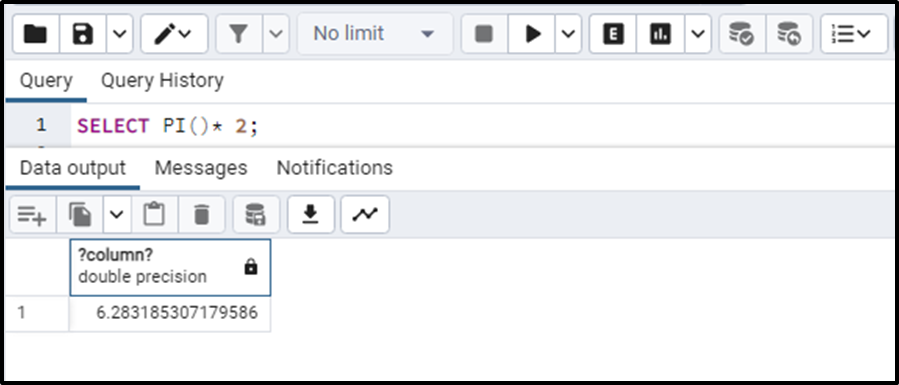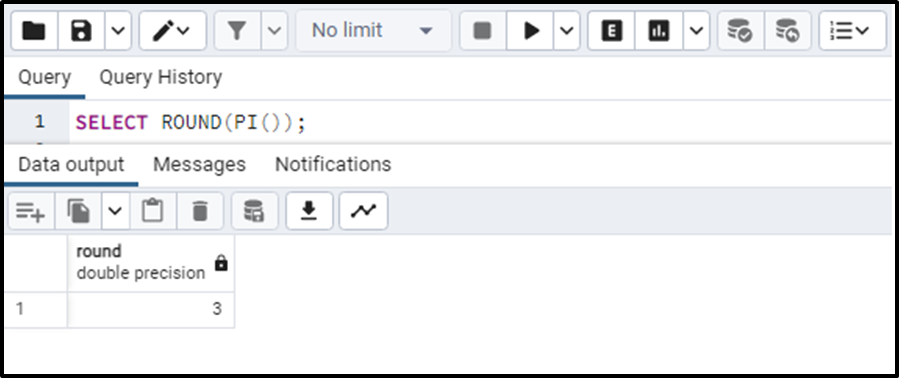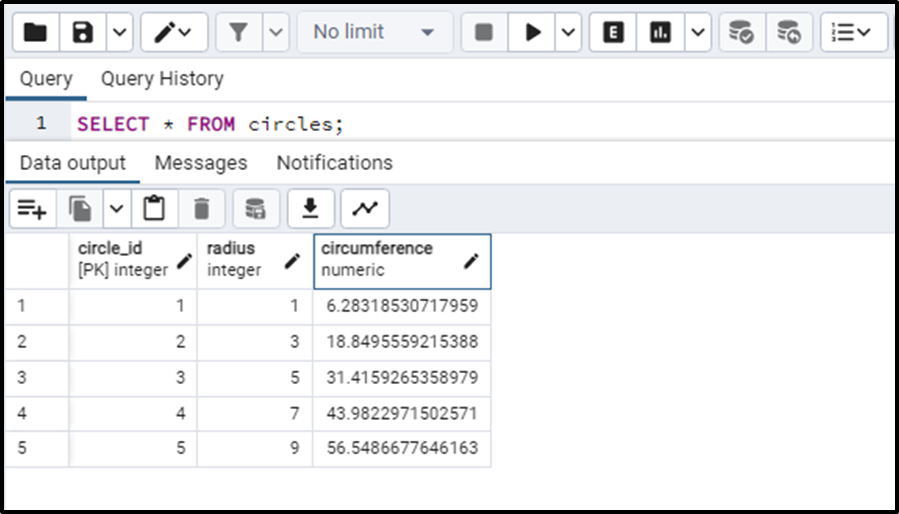##### How Does the pi() Function Work in PostgreSQL?

The pi() function is one of the mathematical functions that is frequently used in PostgreSQL. This function returns the mathematical constant value of the π “pi”. The π is widely used in mathematical calculations and we can also use the mathematical value of π in Postgres by using this pi() function. In this blog, we will use the pi() function and understand how it works.

How Does the pi() Function Work in PostgreSQL?

The pi() function gives the mathematical constant value of π. The syntax of this function is:

`SELECT PI();`

The return type of this function is the DOUBLE PRECISION data type. Let’s execute the pi() function to see what it returns:We can observe that the pi() function has returned the mathematical constant value of π and the data type returned by this function is DOUBLE PRECISION.

We can use it in expressions as well. Let’s see how.

Example 1: pi() Function in PostgreSQL

We can use the pi() function in expressions for example we can execute the following expression to get the desired output:

`SELECT PI()* 2;`

In the above expression, we have multiplied the constant value of π by 2. This will give the following output:This is how we can use the pi() function in any expression.

Example 2: Applying Functions on pi() Function in PostgreSQL

We can apply some other function to the pi() function. Like, in the below query, we will be applying the ROUND() function to the pi() function.

`SELECT ROUND(PI());`

The query will return the rounded value of the π as an output.Similarly, we can apply the CEILING() and FLOOR() functions on the pi() function like this:

```SELECT
CEILING(PI()),
FLOOR(PI());```

The above query gives the ceiling and the floor value of the π in output.

In this simple way, we can apply functions on the pi() function.

Example 3: Using PI() Function to Calculate the Circumference of Circle

Another significant use case of the pi() function is calculating the circumference of circles. Let’s create the following table in which we will be calculating the circumference of the circle using the generated columns. The query can be written as:

```CREATE TABLE circles (
circle_id SERIAL PRIMARY KEY,
circumference DECIMAL
GENERATED ALWAYS AS (2* PI()* Radius) STORED
);```

The query will successfully create the table. Now if we want to insert some values into the table, we will write the following query.

```INSERT INTO circles(Radius)
VALUES(1),(3),(5),(7),(9);```

The values will be inserted in the “circles” table. The values will also be automatically inserted in the ”circumference” column because it is a generated column and the value of each row is computed by the expression provided i.e. “2* PI()* radius”. Now we will run the SELECT statement to get the table.

`SELECT * FROM circles;`

The output of this query is:In this way, we can use the pi() function in a Postgres table to calculate the circumference of circles. There can be more use cases to apply the function in any table.

This was all about the basic functioning of the pi() function in Postgres.

Conclusion

Use the pi() function in PostgreSQL to get the mathematical constant value of π. We can use this function in different mathematical expressions where we need to use the value of π. In this article, we understood the workings of the pi() function with different examples and use cases.